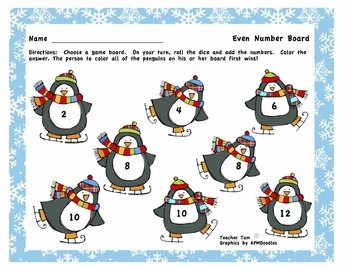DID YOU KNOW:
Seamlessly assign resources as digital activities

Learn how in 5 minutes with a tutorial resource. Try it Now

Learn More# Winter Math Kindergarten 1st 2nd GradeK - 2nd, Homeschool
Subjects
Standards
Resource Type
Formats Included
• PDF
Pages
28 pages

### Description

Kindergarten Math Centers Winter | Kindergarten Math Games Winter . These dice addition and subtraction games help students practice facts up to 12, as well as adding and subtracting 10 from 2-digit numbers. The set includes 12 different games in both full color and blackline versions. The colorful versions can be laminated for repeated use. The games work well in centers, as partner games within a large group, or as take home practice.

Two of the games cover addition facts to 12. Another game has students adding doubles. Four more games have students subtracting quantities from 9, 10, 11, and 12. Two games are more difficult, asking students to add 2 numbers and subtract a third. The most difficult games ask students to make 2-digit numbers, then add or subtract 10.

These games address the following CCSS*:

K.CC.A.2, K.CC.B.4, K.OA.A.4, K.OA.A.5, and K.NBT.A.1

1.OA.B.4, 1.OA.C.5, 1.OA.D.8, 1.NBT.B.2.B, 1.NBT.C.4, and 1.NBT.C.5

2.OA.B.2 and 2.NBT.B.5

For more WINTER MATH fun . . .

Total Pages
28 pages
N/A
Teaching Duration
1 Year
Report this Resource to TpT
Reported resources will be reviewed by our team. Report this resource to let us know if this resource violates TpT’s content guidelines.

### Standards

to see state-specific standards (only available in the US).
Fluently add and subtract within 20 using mental strategies. By end of Grade 2, know from memory all sums of two one-digit numbers.
Determine the unknown whole number in an addition or subtraction equation relating three whole numbers. For example, determine the unknown number that makes the equation true in each of the equations 8 + ? = 11, 5 = ▯ - 3, 6 + 6 = ▯.
Relate counting to addition and subtraction (e.g., by counting on 2 to add 2).
Understand subtraction as an unknown-addend problem. For example, subtract 10 – 8 by finding the number that makes 10 when added to 8.
For any number from 1 to 9, find the number that makes 10 when added to the given number, e.g., by using objects or drawings, and record the answer with a drawing or equation.